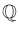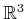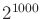#### Lower bounds for motion planning

The general motion planning problem, Formulation 4.1, was shown in 1979 to be PSPACE-hard by Reif . In fact, the problem was restricted to polyhedral obstacles and a finite number of polyhedral robot bodies attached by spherical joints. The coordinates of all polyhedra are assumed to be in(this enables a finite-length string encoding of the problem instance). The proof introduces a fascinating motion planning instance that involves many attached, dangling robot parts that must work their way through a complicated system of tunnels, which together simulates the operation of a symmetric Turing machine. Canny later established that the problem in Formulation 4.1 (expressed using polynomials that have rational coefficients) lies in PSPACE . Therefore, the general motion planning problem is PSPACE-complete.

Many other lower bounds have been shown for a variety of planning problems. One famous example is the Warehouseman's problem shown in Figure 6.41. This problem involves a finite number of translating, axis-aligned rectangles in a rectangular world. It was shown in  to be PSPACE-hard. This example is a beautiful illustration of how such a deceptively simple problem formulation can lead to such a high lower bound. More recently, it was even shown that planning for Sokoban, which is a warehouseman's problem on a discrete 2D grid, is also PSPACE-hard . Other general motion planning problems that were shown to be PSPACE-hard include motion planning for a chain of bodies in the plane [460,490] and motion planning for a chain of bodies among polyhedral obstacles in. Many lower bounds have been established for a variety of extensions and variations of the general motion planning problem. For example, in  it was established that a certain form of planning under uncertainty for a robot in a 3D polyhedral environment is NEXPTIME-hard, which is harder than any of the classes shown in Figure 6.40; the hardest problems in this NEXPTIME are believed to require doubly exponential time to solve.

The lower bound or hardness results depend significantly on the precise representation of the problem. For example, it is possible to make problems look easier by making instance encodings that are exponentially longer than they should be. The running time or space required is expressed in terms of, the input size. If the motion planning problem instances are encoded with exponentially more bits than necessary, then a language that belongs to P is obtained. As long as the instance encoding is within a polynomial factor of the optimal encoding (this can be made precise using Kolmogorov complexity ), then this bizarre behavior is avoided. Another important part of the representation is to pay attention to how parameters in the problem formulation can vary. We can redefine motion planning to be all instances for which the dimension ofis never greater than. The number of dimensions is sufficiently large for virtually any application. The resulting language for this problem belongs to P because cylindrical algebraic decomposition and Canny's algorithm can solve any motion planning problem in polynomial time. Why? This is because now the dimension parameter in the time-complexity expressions can be replaced by, which is a constant. This formally implies that an efficient algorithm is already known for any motion planning problem that we would ever care about. This implication has no practical value, however. Thus, be very careful when interpreting theoretical bounds.

The lower bounds may appear discouraging. There are two general directions to go from here. One is to weaken the requirements and tolerate algorithms that yield some kind of resolution completeness or probabilistic completeness. This approach was taken in Chapter 5 and leads to many efficient algorithms. Another direction is to define narrower problems that do not include the bizarre constructions that lead to bad lower bounds. For the narrower problems, it may be possible to design interesting, efficient algorithms. This approach was taken for the methods in Sections 6.2 and 6.3. In Section 6.5.3, upper bounds for some algorithms that address these narrower problems will be presented, along with bounds for the general motion planning algorithms. Several of the upper bounds involve Davenport-Schinzel sequences, which are therefore covered next.

Steven M LaValle 2020-08-14